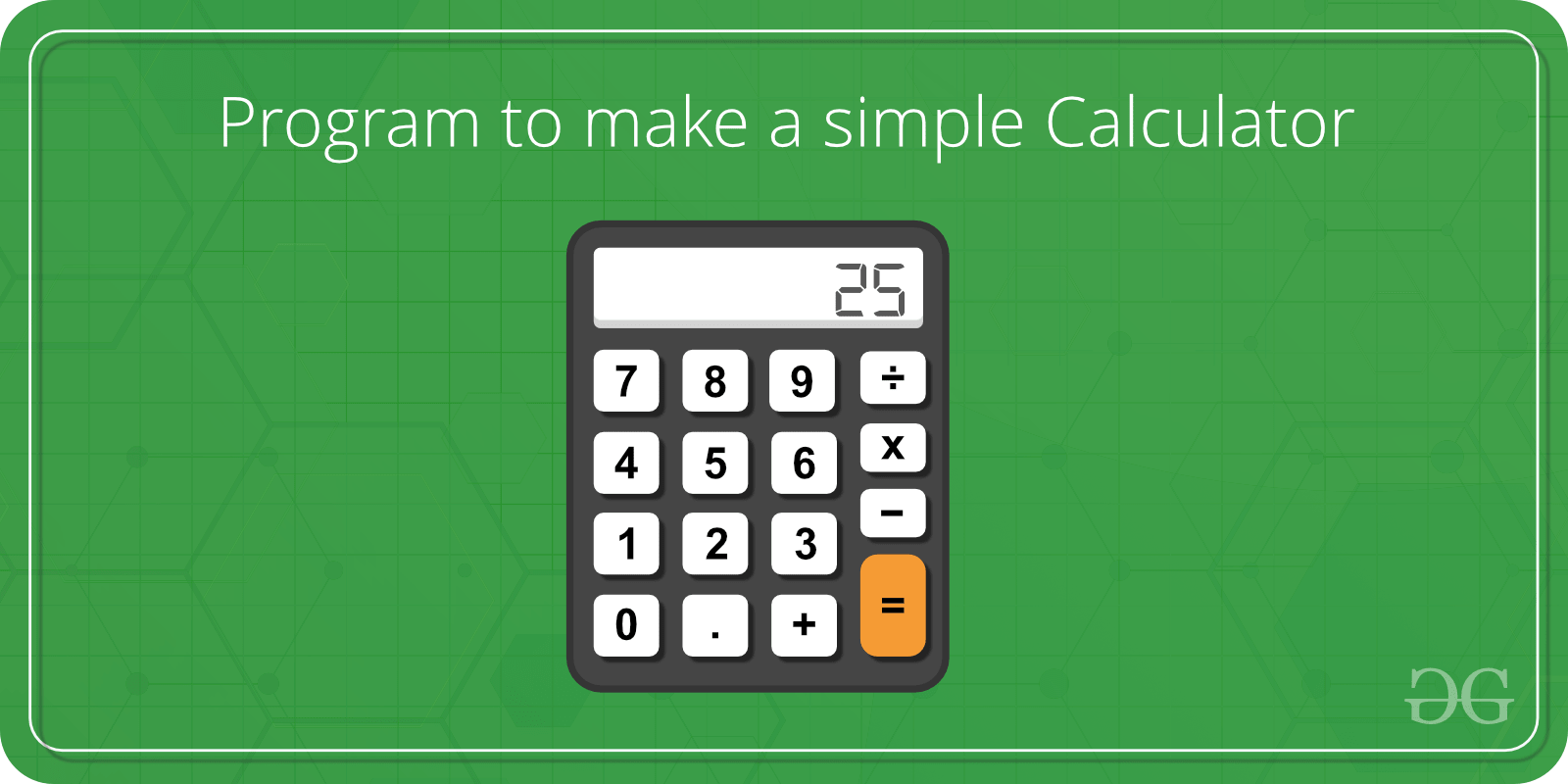# Menu Driven C++ Program for a Simple Calculator

Problem Statement:
Write a menu-driven program using the Switch case to calculate the following:

2. Difference between two numbers
3. Product of two numbers
4. Division of two numbers
5. HCF of two numbers
6. LCM of two numbersExamples:

```Input: num1 = 5, num2 = 7, choice = 1
Output: Sum is 12

Input: num1 = 5, num2 = 7, choice = 5
Output: GCD is 1
```

## Recommended: Please try your approach on {IDE} first, before moving on to the solution.

Implementation:

 `// C++ program to illustrate ` `// Menu-Driven program using Switch-case ` ` `  `#include ` `using` `namespace` `std; ` ` `  `// Function to display the menu ` `void` `menu() ` `{ ` `    ``cout << ``"Press 1 to calculate Sum of Numbers\n"``; ` `    ``cout << ``"Press 2 to calculate Difference of Numbers\n"``; ` `    ``cout << ``"Press 3 to calculate Product of numbers\n"``; ` `    ``cout << ``"Press 4 to calculate Division of numbers\n"``; ` `    ``cout << ``"Press 5 to calculate HCF of numbers\n"``; ` `    ``cout << ``"Press 6 to calculate LCM of numbers\n"``; ` `    ``cout << ``"Press 7 to exit\n"``; ` `} ` ` `  `// Function to calculate and display the result ` `void` `result(``int` `choice, ``int` `a, ``int` `b) ` `{ ` ` `  `    ``// Display the result ` `    ``switch` `(choice) { ` `    ``case` `1: { ` `        ``cout << ``"Sum is "` `<< (a + b) << ``"\n"``; ` `        ``break``; ` `    ``} ` `    ``case` `2: { ` `        ``cout << ``"Difference is "` `<< (a - b) << ``"\n"``; ` `        ``break``; ` `    ``} ` `    ``case` `3: { ` `        ``cout << ``"Product is "` `<< (a * b) << ``"\n"``; ` `        ``break``; ` `    ``} ` `    ``case` `4: { ` `        ``cout << ``"Division is "` `<< (a / b) << ``"\n"``; ` `        ``break``; ` `    ``} ` `    ``case` `5: { ` `        ``cout << ``"GCD is "` `<< __gcd(a, b) << ``"\n"``; ` `        ``break``; ` `    ``} ` `    ``case` `6: { ` `        ``cout << ``"LCM is "` `             ``<< ((a * b) / __gcd(a, b)) ` `             ``<< ``"\n"``; ` `        ``break``; ` `    ``} ` `    ``case` `7: { ` `        ``cout << ``"Thank you\n"``; ` `        ``break``; ` `    ``} ` `    ``default``: ` `        ``printf``(``"Wrong Input\n"``); ` `    ``} ` `} ` ` `  `int` `main() ` `{ ` ` `  `    ``// Get the two numbers ` `    ``int` `a = 5, b = 7; ` ` `  `    ``int` `choice, res; ` ` `  `    ``// Display the menu ` `    ``menu(); ` ` `  `    ``// Enter the choice ` `    ``cout << ``"Enter your choice:\n"``; ` `    ``choice = 1; ` `    ``cout << ``"Choice is "` `<< choice << endl; ` ` `  `    ``// Display the result ` `    ``// according to the choice ` `    ``result(choice, a, b); ` ` `  `    ``return` `0; ` `} `

Output:

```Press 1 to calculate Sum of Numbers
Press 2 to calculate Difference of Numbers
Press 3 to calculate Product of numbers
Press 4 to calculate Division of numbers
Press 5 to calculate HCF of numbers
Press 6 to calculate LCM of numbers
Press 7 to exit
Choice is 1
Sum is 12
```

Time Complexity: O(n).

GeeksforGeeks has prepared a complete interview preparation course with premium videos, theory, practice problems, TA support and many more features. Please refer Placement 100 for details

My Personal Notes arrow_drop_upCheck out this Author's contributed articles.

If you like GeeksforGeeks and would like to contribute, you can also write an article using contribute.geeksforgeeks.org or mail your article to contribute@geeksforgeeks.org. See your article appearing on the GeeksforGeeks main page and help other Geeks.

Please Improve this article if you find anything incorrect by clicking on the "Improve Article" button below.